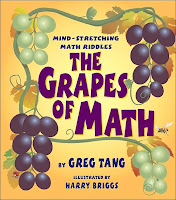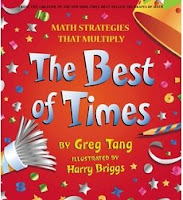Thursday, March 19, 2009

Grapes of MathLast September, a fellow blogger turned me onto Greg Tang books for dynamic thinking about math, and I blogged an update on our progress in November. So far, we have slowly worked our way through the following books: Best of Times, Math Appeal, and Math for All Seasons. We are looking forward to working through the five books we have not read yet.Right now, we are starting Grapes Of Math and I thought it high-time to share what we are doing with these deceptively simple, elementary-school-level books. Basically, you read the poem and use the clue to count items in a picture. In this counting exercise, the clue is "Never fear, I have a hunch there is a match for every bunch." When you match a bunch of seven purple grapes with three green grapes, you get ten. Five matches of ten grapes yields the answer ten.Even though Pamela is near the end of sixth grade math, these books are valuable because she is learning to group and count items in unique ways that make them easier to count. They guide you to count dynamically! Rather than get wrapped up in the poem in a static way, we adopt various strategies for counting the items. Pamela and I take turns showing each other a new way to count. We usually do three patterns each. Today, two interesting things happened. Pamela made a mistake in mentally adding and ended up with the wrong answer: 52. Rather than telling her she was wrong, I counted and got the right answer: 50. Then, we observed our answers were different and kept trying various counting strategies. From that point on our answers were always the same: 50. Pamela surprised me in her last strategy: she counted them individually and silently! The video clip shows a typical session with the book.Best of Times gave us a fresh look at multiplication tables. There was nothing new about x 0, x 1, and x 10, but the way the author explored other numbers was original for this old dog (you know, ME). These unique ways of multiplying captured Pamela's imagination, and I plan to apply them when we are doing mental math while we shop at our favorite grocery stores!

x 2 - Double the number by adding it to itself:
37 x 2 = 37 + 37 = 74

x 3 - Triple the number by doubling it first and adding the double to itself:
45 x 3 = (45 + 45) + 45 = 90 + 45 = 135

x 4 - Double the double of the number (and I bet you can guess times eight now):
42 x 4 = (42 + 42) + (42 + 42) = 84 + 84 = 168

x 5 - Find x 10 and cut in half:
57 x 5 = (57 x 10) / 2 = 570 / 2 = 285

Here's a dynamic newsflash, not in the book: add the double to the triple:
57 x 5 = (57 + 57) + [(57 + 57) + 57]
= 114 + (114 + 57) = 114 + 171 = 285

Aside, not in the book: why not double the double and add it to itself?
57 x 5 = (57 + 57) + (57 + 57) + 57
= (114 + 114) + 57 = 228 + 57 = 285

x 6 - Triple the double (or vice versa):
28 x 6 = [(28 + 28) + (28 + 28)] + (28 + 28)
= (56 + 56) + 56 = 112 + 56 = 168

28 x 6 = [(28 x 10) / 2] + 28
= (280 / 2 ) + 28 = 140 + 28 = 168

x 7 - Add x 5 and x 2 (or figure out your own variation):
82 x 7 = [(82 x 10) / 2] + (82 + 82)
= (820 / 2) + 164 = 410 + 164 = 574

x 8 - Double the double of double of the number:
65 x 8 = [(65 + 65) + (65 + 65)] + [(65 + 65) + (65 + 65)]
= (130 + 130) + (130 + 130) = 260 + 260 = 520

x 9 - Figure out x 10 and subtract the number:
19 x 9 = 19 x 10 - 19 = 190 - 19 = 171Anonymous said...

Awesome! :-)

argsmommy said...

Those look wonderful! Thanks for posting.

Kellie

walking said...

Thanks, Mama Monkey, because you were the one who got me into these books (and my consultant is using them for her NT son right now)!!!!